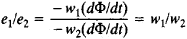# Turn Ratio

## Turn Ratio

(also turns ratio), the ratio of the electromotive forces (emf’s) induced in the primary and secondary windings of a transformer by the mutual magnetic flux, that is, the flux in the magnetic circuit. It is equal towhere e1 and e2 are the emf’s in the primary and secondary transformer windings, w1 and w2 are the numbers of turns in the primary and secondary windings, and Φ is the mutual magnetic flux. In practice the turn ratio is defined as the ratio of the rated supply voltage of the primary winding to the voltage of the open secondary winding, and the error caused by the difference between the emf and the voltage of the primary winding is neglected.

References in periodicals archive ?
To prosper voltage gain ratio the coupled inductor secondary side winding are connected in series and operate as voltage source in proportion to turn ratio.
Voltage regulation is performed by varying the transformer turn ratio.
Source voltage 30V Battery terminal voltage 24 V Transformer turn ratio 3:1 Inductor 13.
The value of the turn ratio is approximately determined by n = [aquare root of [Z.
The MC-6 has a lower rate of descent, lower opening shock, reduced canopy damage, a better turn ratio and a better glide ratio than the MC1-1C.
Marketing to a 70-80 percent turn ratio is extremely tough.
Precision machined from aluminum, goniometric arcs are etched with white on black vernier scales and rotation stage has 45:1 turn ratio.
Limited Tenders are invited for Repairing Of Three Ph Transformer Turn Ratio Kit Ttr At Ntpc-Tanda.
3-phase transformer turns ratio test set; turn ratio range: 0.

Site: Follow: Share:
Open / Close